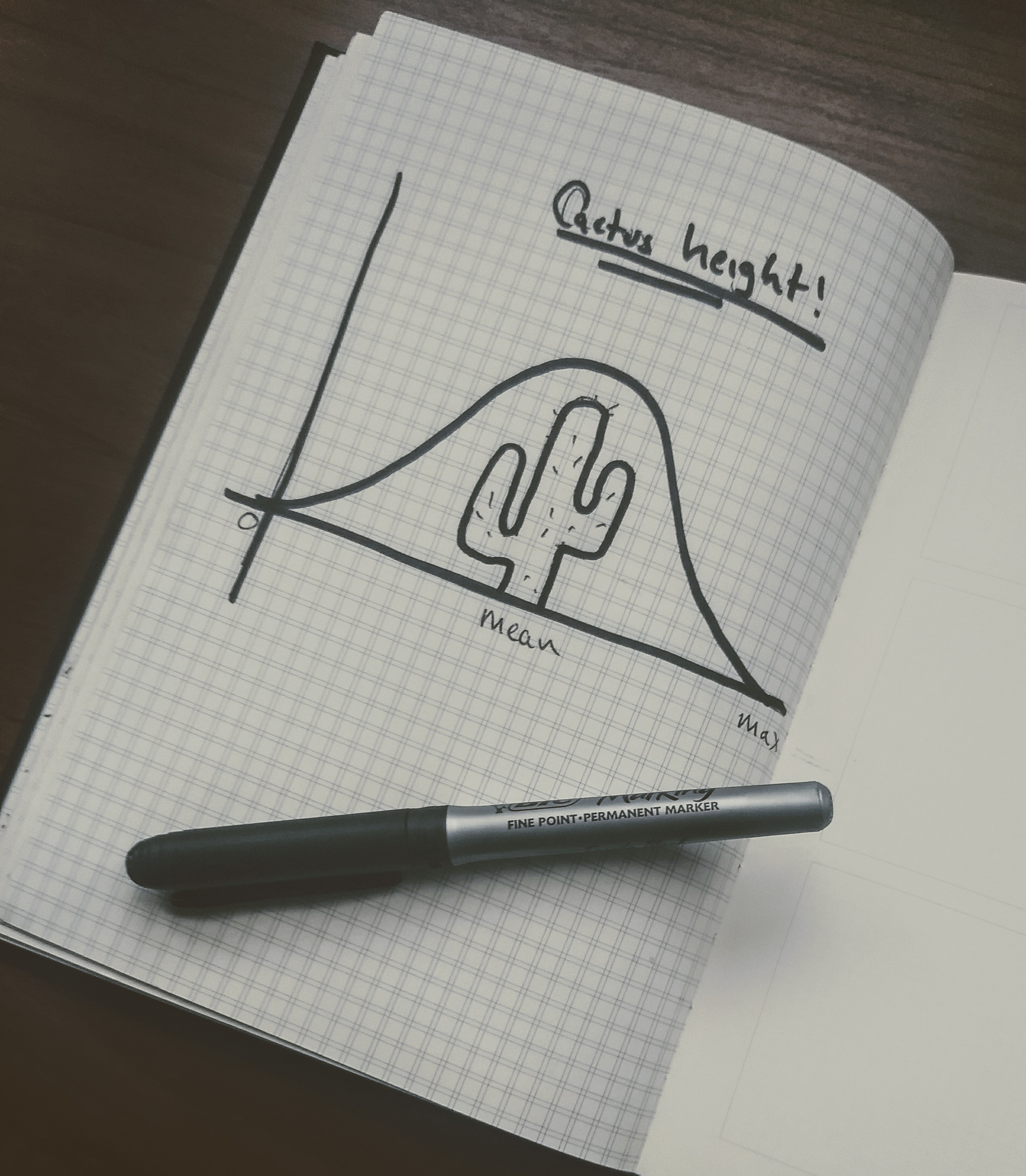## Normal Distribution : Taming the Random

2 minutes
Contact me

In post one I looked a random walker, a small dot that moved across the screen based on a number between 0 - 3.

Although It worked, It didn't move in a way that seemed natural. It was, well, too random.

Wouldn't it be great to generate sets of numbers that seem more naturally distributed.

Well, it just so happens that is possible to do so.

## Gaussian Distribution

In reality things are not totally random.

Height is a good example of this.

Say we are looking at the heights of cacti.

``````       `""',
;' ` ;
;`,',;
;' ` ;
,,,  ;`,',;
;,` ; ;' ` ;   ,',
;`,'; ;`,',;  ;,' ;
;',`; ;` ' ; ;`'`';
;` '',''` `,',`',;
`''`'; ', ;`'`'
;' `';
;` ' ;
;' `';
;` ' ;
; ',';
;,' ';
dwb\|/\|/;\|/``````

With the random approach cactus height between 1 - 100 would eventually end up like this:

``````|
|
|_____________________
|
|
|_____________________
0            100 ``````

That's because each number has an equal chance of being picked.

That doesn't really reflect reality as there would be more cacti around the middle or the mean like so:Apparently you can enhance the look of a graph by putting a pen across the page you just drew on.

That's more like it, a lot more around the mean and a few outliers.

This would be an example of a normal distribution, a mean of 50 and a standard deviation of 2.

How to calculate standard deviation see figure 1.4.

How does standard deviation look:

The larger the standard deviation the shallower the curve, in the pictures above the left image would have a lower standard deviation that the right picture.

Let's apply these ideas to code:

See the Pen Normal Distribution by Adam Harpur (@harps116) on CodePen.

In this example I generated a random sample of 10,000 numbers between 1 - 10 with a mean of 5 and standard deviation of 1. This set is then plotted on a graph. As you can see our chart behaves as expected with most of the numbers hovering around the average of 5 and less around 1 and 10.

I added some comments and references to the code so it's worth checking out if you'd like see how everything works together. You can also open your console ( ctrl + shift + i and click the console tab to see the logs ).

Feel free to change the labelled values around to see how the chart grows and shrinks. Decreasing the standard deviation will create more numbers closer to the mean. Increasing the standard deviation will create more numbers towards the max high and low.

Thanks for reading && happy coding!

Check out Daniel Shiffman's great series on the Nature of Code, this 100 days of learning is a great excuse for me to finally work through the lessons and take some notes as I go.